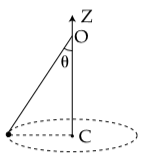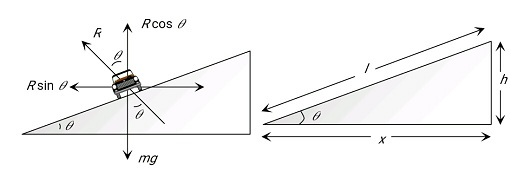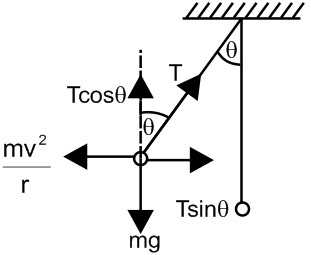# A conical pendulum of length 1 m makes an anglew.r.t. Z-axis and moves in a circle in the XY plane.  The radius of the circle is 0.4 m and its center is vertically below O.  The speed of the pendulum, in its circular path, will be : (Take g=10 ms−2)Option 1) 0.4 m/s Option 2) 4 m/s Option 3)  0.2 m/s Option 4)  2 m/s

As we learnt in

If friction is also present in banking of road -angle of banking

coefficient of friction

V = velocity

- wherein

Maximum speed on a banked frictional road(i)

(ii)

Correct option is 4.

Option 1)

0.4 m/s

This is an incorrect option.

Option 2)

4 m/s

This is an incorrect option.

Option 3)

0.2 m/s

This is an incorrect option.

Option 4)

2 m/s

This is the correct option.

Exams
Articles
Questions